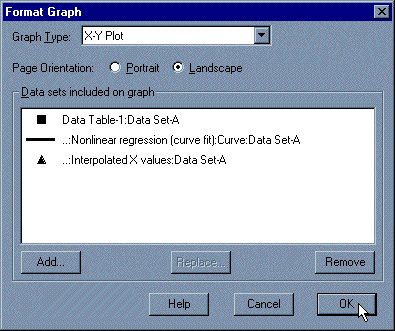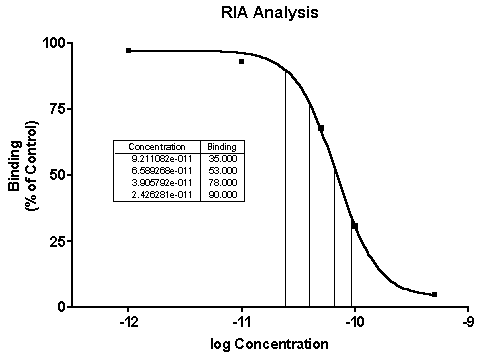KNOWLEDGEBASE - ARTICLE #1752

# Prism 3 -- Analyzing RIA and ELISA Data

Step-by-Step Examples:
Analyzing RIA and ELISA Data using Prism 3

Analyzing radioimmunoassay (RIA) or an enzyme-linked immunosorbent assay (ELISA) data is a two-step process:

• Prepare and assay a set of "known" samples, i.e., samples that contain the substance of interest in amounts that you choose and which span the range of concentrations that you expect to be present in your "unknown" samples.  The quantity that you measure (radioactivity, optical density, or luminescence, for example) is related graphically to sample concentration to produce a standard curve.
• Assay the "unknown" samples. Then, for each measurement obtained, use the standard curve to find the corresponding substance concentration. Graphically, this amounts to finding the X-axis value (concentration) for a given Y-axis value (measured quantity).

Prism automates the process of producing a standard curve and interpolating unknown concentrations from it. Follow these steps:

Enter the Data

In the Welcome to Prism dialog box, select Create a new project and Work independently. Choose to format the X column as Numbers and to format the Y column for the number of replicates in your data. For this example, choose A single column of values.

RIA/ELISA data often span such large concentration ranges that concentrations are plotted as their logarithms. We'll follow that convention here and let Prism compute the logarithms for us.

Enter data for the standard curve. For our example, enter "known" sample concentrations into the X column, rows 1-6, and the corresponding assay results into the Y column. Sometimes you'll want to include data from a zero-concentration sample. Since our data will be graphed on a semi-log plot and it's impossible to plot the log of 0, we approximate the zero-concentration point in row 1 with an X coordinate of 1.0e-014, two log units below the lowest non-zero X value. Including this data point will help to define the top of the fitted curve more accurately.

Just below the standard curve values, enter the assay results (Y values, rows 7-10) for the "unknown" samples, leaving the corresponding X cells blank. Later, Prism will fit the standard curve and then report the unknown concentrations using that curve.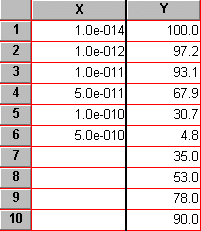Transform the data

To convert the values in the X column to their logarithms, click Analyze. Choose Built-in analysis. From the Data manipulations category, select Transforms. Choose to analyze All data sets.

In the Parameters: Transformations dialog, choose to Transform X values using X=Log(X). The selections are shown below.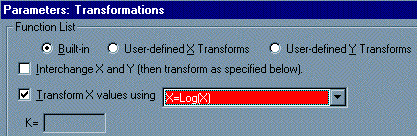At the bottom of this same dialog box, choose to Create a new graph of the results. Click OK. Prism displays a new Results sheet with the log-transformed X values.

Fit the Curve

Click on the Analyze button. Choose to Analyze the results table you are looking at.

From the Curves & Regression category, select Nonlinear regression (curve fit).

In the Parameters: Nonlinear regression dialog box, choose Classic equations.  Select Sigmoidal dose-response (variable slope) for our example, although you may wish to experiment with other equations. Since we want our unknown concentrations to be provided, check the box labeled Unknowns from the standard curve. Click Output, and under the Range of X values category, de-select Choose min. X value automatically. For Minimum X=, enter -12 (we are truncating the curve at the lowest non-zero X coordinate).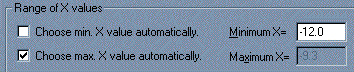When you click OK, twice, to leave the nonlinear regression parameters dialog, Prism performs the fit and creates a results sheet.

View the Results

Prism displays the results of nonlinear regression on pages called views. The default view shows the defining parameters for the curve of best fit. For a routine RIA, you may not care too much about the information contained in this page.

Locate the drop-down box labeled "View" in the third row of the tool bar. Switch from the default view (the table of results) to Interpolated X values (in early Prism releases, this was "Standard curve X from Y"). Prism reports the corresponding X value for each unpaired Y value on your data sheet. These values are expressed as logarithms of concentration.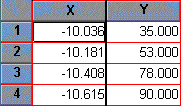Change from Log of Concentration to Concentration

Although it's generally easiest to plot and analyze semi-logarithmic RIA/ELISA data, we'd like to read our unknown concentrations directly, not as logarithms.

Click on the Analyze button, and choose Analyze the results table you are looking at.

From the Data Manipulations category, select Transforms and make sure that the table listed in the window below is the "...Interpolated X values" (or ...Standard Curve X from Y).

In the Parameters: Transformations dialog, check the box for Transform X values using... Select the equation X=10^X from the drop-down list.

Click on OK. Prism creates another results sheet, on which the X values are now expressed as concentration.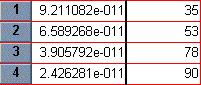Prism's automatic graph includes the data from the data sheet and the curve. To add the "unknowns" to the graph:

Switch to the Graphs section of your project.

Click on the Change button and then select Data on Graph.

The dialog box shows all data and results tables that are represented on the graph. Click on the Add button.
From the drop-down list at the top of the Add Data Sets to Graph dialog box, select Nonlinear regression (curve fit): Interpolated X values (or ...Standard Curve X from Y).  Click on Add, then Close. The list of data sets included on the graph should now look like the window below.